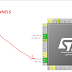I have already posted articles about ADC in STM32 using PollforConersionInterrupt and DMA methods. But in all those articles, I used single channel of the ADC. So today We will see How to read multichannel ADC in STM32.
The process remains same as using in single channel, with some addons though. Let’s get into it
First we need to set up the channels, we want to read, under ADC. Here I will read Channels 0, 1 and internal temperature sensor (16).

## 1.) USING PollforConversion Method

In the ADC configuration, Enable SCAN CONVERSION and Continuous Conversion, If you don’t know what they are, please go to the links in the first paragraph.

Setup is complete now.. Just open the project and follow along.
First we need to define some variables to store the values of the ADC
``uint32_t adc1, adc2, adc3, temperature;``

The process is simple here. All you need to do is start ADC -> read adc1 value -> read adc2 value -> read adc3 value and stop ADC. So here its goes
``````HAL_ADC_Start(&hadc1);

temperature = (((temp_val*vsense)-.76)/.0025)+25;

HAL_Delay (200);

``````

The value returned by channel 16 need to be converted inorder to get the temperature. The formula and the relative values are given in the datasheet. I am using NUCLEOF401RE and the following is extracted from the datasheet.

So we can define Vsense as 3.3/1023 because we are using 10 bit resolution for the ADC.
``````float vsense = 3.3/1023;
``````

## 2.) USING DMA METHOD

To use DMA, we need to enable the DMA continuous request in the ADC configuration. Also enable the interrupt and setup the DMA as shown below->

Generate the project and open it.

``````uint32_t adc, buffer, temperature;  // define variables
float vsense = 3.3/1023;
``````

Now we have to write a conversion complete function. It will be called whenever the conversion of the ADC values is completed.

``````void HAL_ADC_ConvCpltCallback(ADC_HandleTypeDef* hadc)
{
for (int i =0; i<3; i++)
{
adc[i] = buffer[i];  // store the values in adc[]
}
}``````
Start the ADC in DMA mode in the main function.
``HAL_ADC_Start_DMA(&hadc1, buffer, 3);``

As to show that it works completely independent, I will blink a LED inside the while loop and the ADC values will be received separately from the while loop.

``````while (1)
{
HAL_GPIO_TogglePin (GPIOA, GPIO_PIN_5);
HAL_Delay (1000);
}
``````
Read multichannel ADC in STM32Reviewed by arun singh on January 28, 2018 Rating: 5